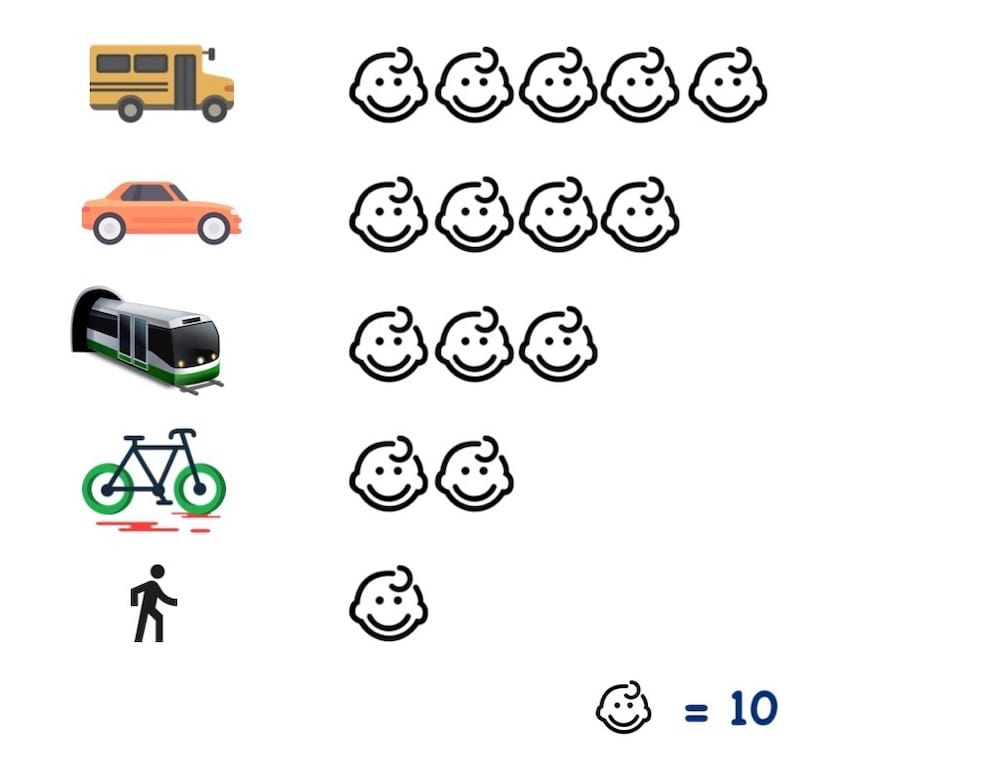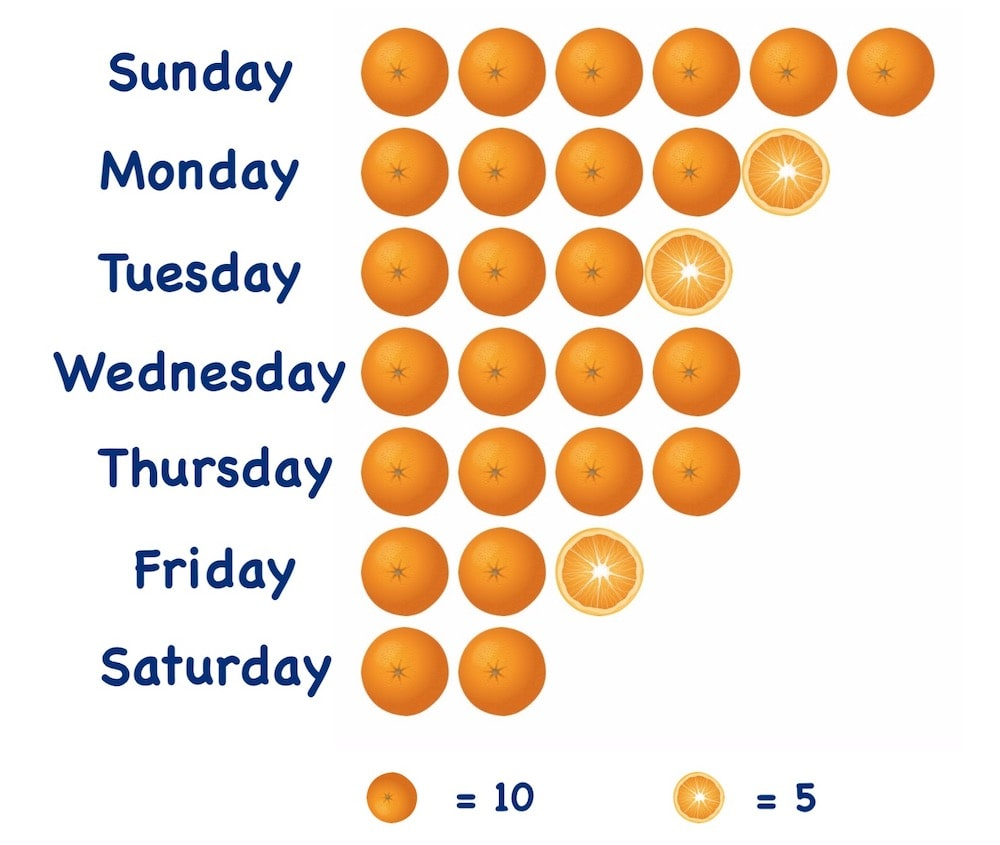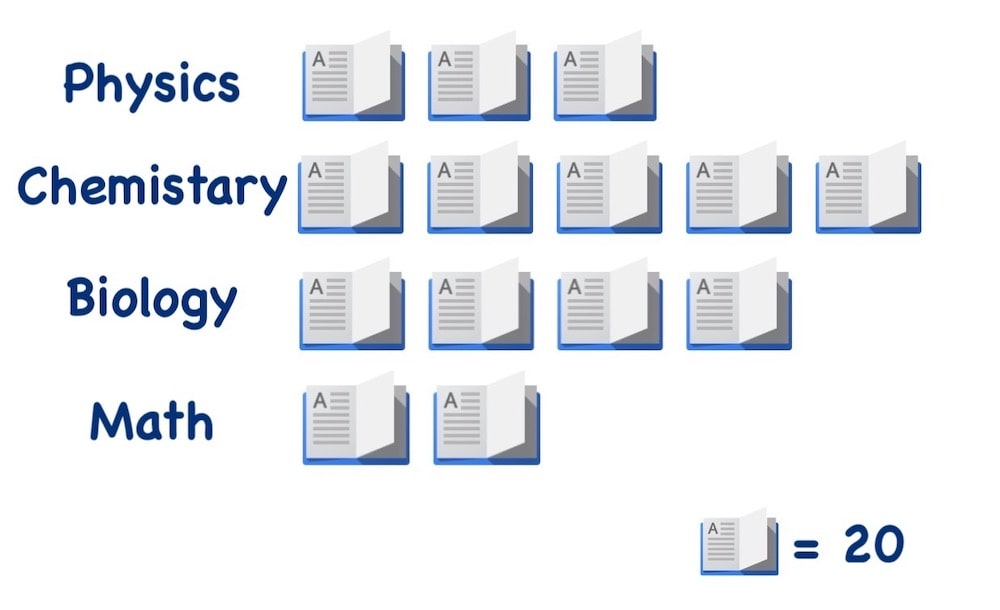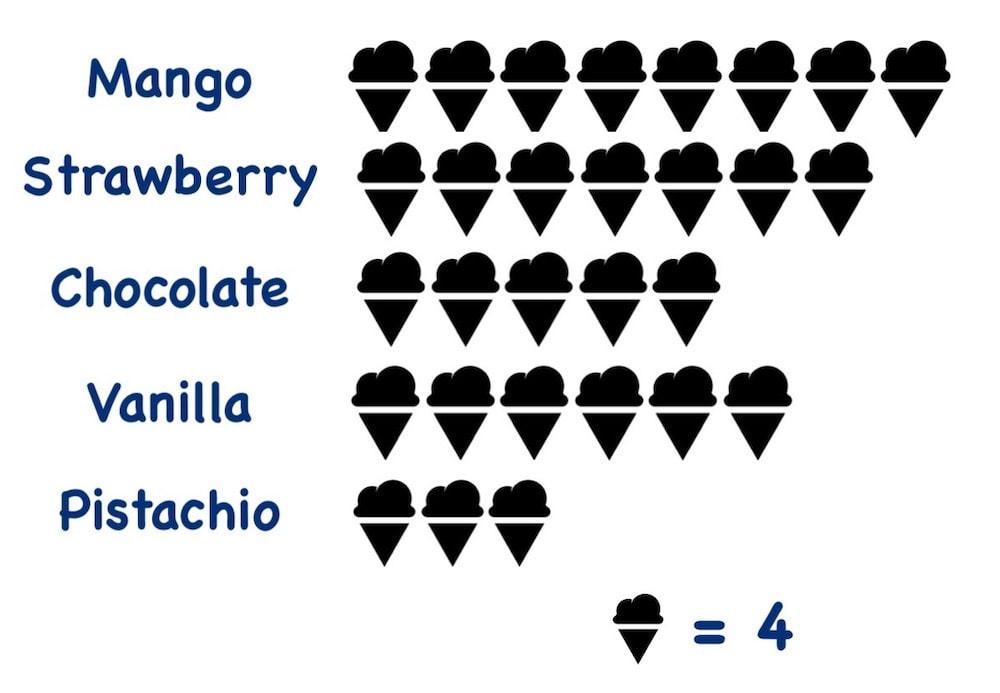# Pictograph Definition, Examples, and Questions

## What is Pictogram or Pictograph?

A Pictograph is also known as Pictogram and some people call it Picto in short. Pictographs are the way to explain things using pictures rather than writing everything in words. For example, you must have seen many road signs that indicate various things. Take an example of the below image that is a road sign and it tells you not to park your car. So wherever you see this sign, you are not supposed to park your car, else you may receive a penalty by traffic police.### Why should we use Pictographs in Education for Kids? How Does it Help?

Kids like images and they make the study more entertaining. That’s one of the reasons many school books include pictographs.

## Using Pictograph in Math

### Example 1: Kids Transport Related Pictograph and Math QuestionsThe picture explains some kids go to school by Bus, some go by Car, some uses Metro Train as Transport, some rides the Bicycle to school, and some Walk to school.

• One Kid icon is equal to 10 kids.

Question: How many kids use Bus as transport?
Answer: 5 x 10 = 50 Kids

Question: How many Kids come by Car?
Answer: 4 x 10 = 40 Kids

Question: How many of them travel by Metro Train?
Answer: 3 x 10 = 30 Kids

Question: Some of the kids use their Bicycle to go to school. Find out how many kids go by Bicycle?
Answer: 2 x 10 = 20 kids

Question: How many kids walk to school?
Answer: 1 x 10 = 10 kids

Question: How many kids study in this school?
Answer: 50 + 40 + 30 + 20 + 10 = 150 kids

Did you learn how a pictograph can help to make many questions? It certainly helps children learn more quickly.

### Example 2: A Pictograph about Orange Seller• 1 orange in the pictograph is equal to 10 oranges and one half is equal to 5 oranges.

What does Pictograph Explain About the Orange Seller?

• The orange seller sells (6 x 10) 60 oranges on Sunday.
• The orange sale of Monday is [(4 x 10) + (1 x 5)] 45 oranges on Monday.
• He sells [(3 x 10) + (1 x 5)] 35 oranges on Tuesday.
• (4 x 10) 40 oranges get sold out on Wednesday.
• The sale of Thursday is also (4 x 10) 40 oranges.
• Friday’s sale is [(2 x 10) + (1 x 5)] 25 oranges.
• Saturday’s sale is (2 x 10) 20 oranges only.

Question: How many oranges do the seller sell in a week?
Answer: 60 + 45 + 35 + 40 + 40 + 25 + 20 = 265 oranges.

Question: What day of the week does the seller sell more oranges?
Answer: Sunday.

### Example 3: A Pictograph about Kids’ Blood Group in a Class• Each blood icon means 2 kids.

The Pictograph explains these facts

• There are (2 x 7) 14 kids in the class whose blood group is A+
• (2 x 5) 10 kids have the blood group B+
• The blood group of (2 x 6) 12 kids is AB+
• (2 x 4) 4 kids’ blood group is O-
• There are (2 x 3) 6 kids whole blood group is O+

Question: How many kids are there in the classroom?
Answer: 14 + 10 + 12 + 4+ 6 = 46 kids.

### Example 4: A Pictograph about Available Books in the Library• Each book icon is equal to 20 books.

What does Pictogram or Pictograph explain?

• The Library has (3 x 20) 60 Physics Books.
• There are (5 x 20) 100 Chemistry Books.
• There are (4 x20) 80 Biology Books in the library.
• You will find only (2 x 20) 40 Math Books in the library.
• Total number of books in the library = 60+100+80+40 = 280 Books.

### Example 5: A Pictogram or Pictograph about Ice cream flavors• 1 ice cream icon is equal to 4 ice creams.

I hope you already know now what ice cream pictograph explains. I will still explain:

• We have 8 x 4 = 32 Mango ice creams
• There are 7 x 4 = 28 Strawberry ice creams
• We got 5 x 4 = 20 Chocolate ice creams
• We have 6 x 4 = 24 Vanilla ice creams
• There are 3 x 4 = 12 Pistachio ice creams
• Total number of ice creams are 32+28+20+24+12 = 116 ice creams